# Uniform Spanning Trees¶

The Uniform measure on Spanning Trees (UST) of a directed connected graph corresponds to a projection DPP with kernel the transfer current matrix of the graph. The later is actually the orthogonal projection matrix onto the row span of the vertex-edge incidence matrix. In fact, one can discard any row of the vertex-edge incidence matrix - note $$A$$ the resulting matrix - to compute $$\mathbf{K}=A^{\top}[AA^{\top}]^{-1}A$$.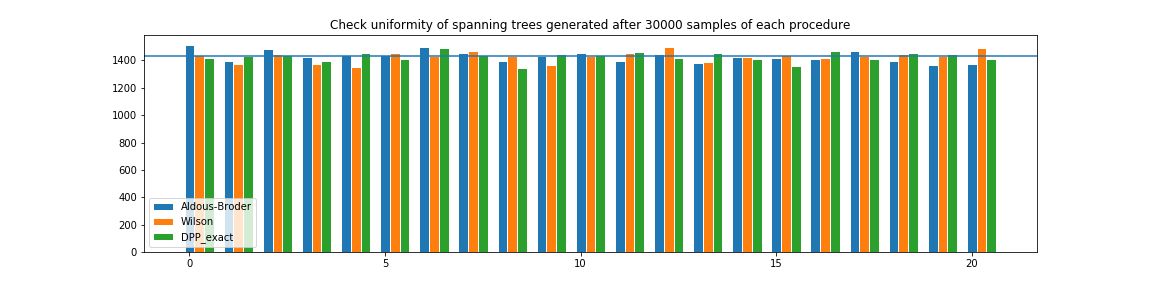from networkx import Graph
from dppy.exotic_dpps import UST

# Build graph
g = Graph()
edges = [(0, 2), (0, 3), (1, 2), (1, 4), (2, 3), (2, 4), (3, 4)]

# Initialize UST object
ust = UST(g)

# Display underlying kernel i.e. transfer current matrix
ust.plot_graph()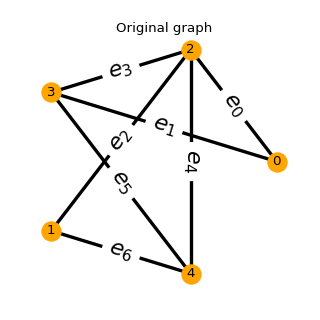# Display underlying kernel i.e. transfer current matrix
ust.plot_kernel()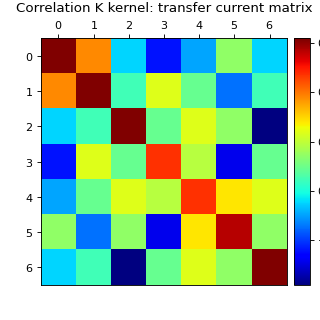# Display some samples
for md in ('Wilson', 'Aldous-Broder', 'GS'):
ust.sample(md)
ust.plot()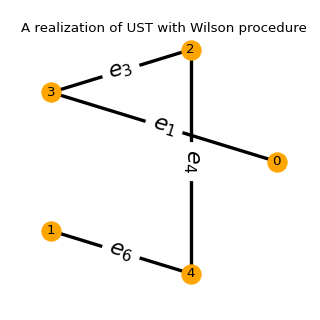Fig. 44 (png, hires.png, pdf)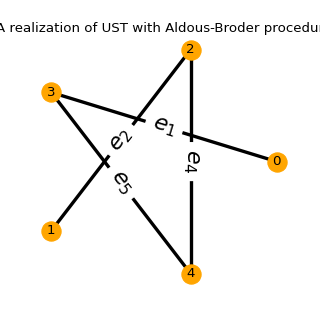Fig. 45 (png, hires.png, pdf)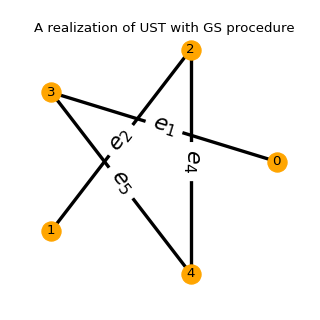Fig. 46 (png, hires.png, pdf)